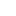• ### Comparing lethal dose ratios using probit regression with arbitrary slopes

分类： 生物学 >> 微生物学 提交时间： 2018-11-26Chengfeng Lei, Xiulian Sun

摘要：Background: Evaluating the toxicity or effectiveness of two or more toxicants in a specific population often requires specialized statistical software to calculate and compare median lethal doses (LD50s). Tests for equality of LD50s using probit regression with parallel slopes have been implemented in many software packages, while tests for cases of arbitrary slopes are not generally available. Methods: In this study, we established probit-log(dose) regression models and solved them by the maximum likelihood method using Microsoft Excel. The z- and χ2-tests were used to assess significance and goodness of fit to the probit regression models, respectively. We calculated the lethal doses (LDs) of the toxicants at different significance levels and their 95% confidence limits (CLs) based on an accurate estimation of log(LD) variances. We further calculated lethal dose ratios and their 95% CLs for two examples without assuming parallel slopes following the method described by Robertson, et al., 2017. Results: We selected representative toxicology datasets from the literature as case studies. For datasets without natural responses in the control group, the slopes, intercepts, χ2 statistics and LDs calculated using our method were identical to those calculated using Polo-Plus and SPSS software, and the 95% CLs of the lethal dose ratios between toxicants were close to hose calculated using Polo-Plus. For datasets that included natural responses in the control group, our results were also close to those calculated using Polo-Plus and SPSS. Conclusion: This procedure yielded accurate estimates of lethal doses and 95% CLs at different significance levels as well as the lethal dose ratios and 95% CLs between two examples. The procedure could be used to assess differences in the toxicities of two examples without the assumption of parallelism between probit-log(dose) regression lines.

同行评议状态:待评议